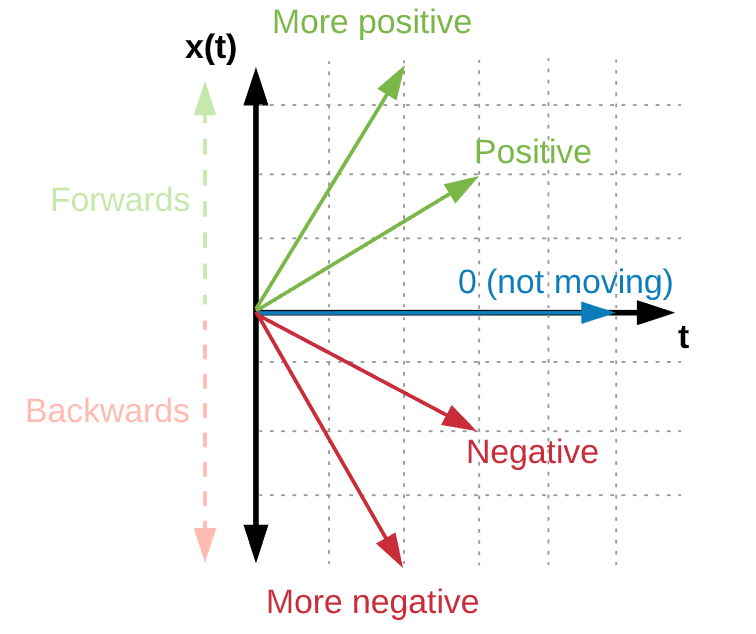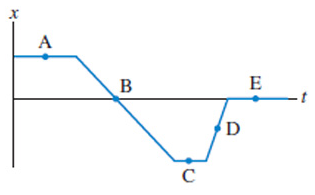# Problem: The figure below shows the position-versus-time graph for a moving object. At which lettered point(s):(a) Is the object moving the slowest?(b) Is the object moving the fastest?(c) Is the object at rest?(d) Is the object moving to the left?

###### FREE Expert Solution

This problem asks us to describe the direction and relative magnitude of the object's velocity at various points based on its position-time graph.

Anytime we're given a position, velocity, or acceleration graph to work with, a PVA diagram like the one below can help remind you of the relationships between the three functions.

$\mathbit{P}\begin{array}{c}{\mathbf{←}}\\ {\mathbf{\to }}\end{array}\underset{{\mathbit{s}}{\mathbit{l}}{\mathbit{o}}{\mathbit{p}}{\mathbit{e}}}{\overset{{\mathbit{a}}{\mathbit{r}}{\mathbit{e}}{\mathbit{a}}}{\mathbit{V}}}\begin{array}{c}{\mathbf{←}}\\ {\mathbf{\to }}\end{array}\mathbit{A}$

Moving left to right, we take the slope of the graph—the slope of a position graph is the velocity

Horizontal lines have zero displacements (Δx) and zero slopes. The diagram below demonstrates how magnitude of a slope varies.(a) We're asked to determine at which point the object is moving the slowest.

87% (208 ratings)###### Problem Details

The figure below shows the position-versus-time graph for a moving object. At which lettered point(s):
(a) Is the object moving the slowest?
(b) Is the object moving the fastest?
(c) Is the object at rest?
(d) Is the object moving to the left?